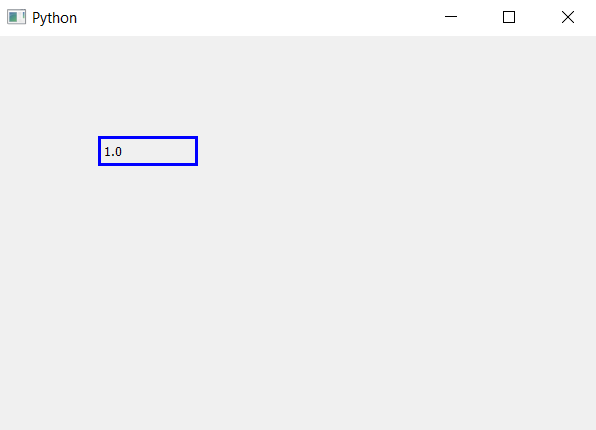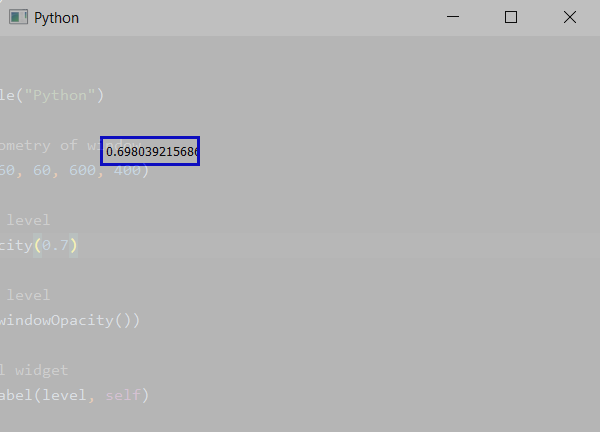# PyQt5 – Get opacity level of the main window | windowOpacity() method

`windowOpacity()` method allows us to see the opacity level of the main window. Opacity is the measure of impenetrability to electromagnetic or other kinds of radiation, especially visible light. In radiative transfer, it describes the absorption and scattering of radiation in a medium, such as a plasma, dielectric, shielding material, glass, etc.

Opacity level ranges from 0.0 to 1.0, where 0.0 means completely transparent and 1.0 means completely opaque, by default it is 1.0 i.e completely opaque.

Syntax : self.windowOpacity()

Argument : It takes no argument.

Return : Returns a float value ranging from 0.0 to 1.0

Example 1:

 `# importing the required libraries ` ` `  `from` `PyQt5.QtCore ``import` `*`  `from` `PyQt5.QtGui ``import` `*`  `from` `PyQt5.QtWidgets ``import` `*`  `import` `sys ` ` `  ` `  `class` `Window(QMainWindow): ` ` `  ` `  `    ``def` `__init__(``self``): ` `        ``super``().__init__() ` ` `  ` `  `        ``# set the title ` `        ``self``.setWindowTitle(``"Python"``) ` ` `  `        ``# setting  the geometry of window ` `        ``self``.setGeometry(``60``, ``60``, ``600``, ``400``) ` ` `  `        ``# getting opacity level ` `        ``level ``=` `str``(``self``.windowOpacity()) ` ` `  `        ``# creating a label widget ` `        ``self``.label_1 ``=` `QLabel(level, ``self``) ` ` `  `        ``# moving position ` `        ``self``.label_1.move(``100``, ``100``) ` ` `  `        ``# setting up the border ` `        ``self``.label_1.setStyleSheet(``"border :3px solid blue;"``) ` ` `  `        ``# show all the widgets ` `        ``self``.show() ` ` `  ` `  `# create pyqt5 app ` `App ``=` `QApplication(sys.argv) ` ` `  `# create the instance of our Window ` `window ``=` `Window() ` `# start the app ` `sys.exit(App.``exec``()) `

Output :Example 2:

 `# importing the required libraries ` ` `  `from` `PyQt5.QtCore ``import` `*`  `from` `PyQt5.QtGui ``import` `*`  `from` `PyQt5.QtWidgets ``import` `*`  `import` `sys ` ` `  ` `  `class` `Window(QMainWindow): ` ` `  ` `  `    ``def` `__init__(``self``): ` `        ``super``().__init__() ` ` `  ` `  `        ``# set the title ` `        ``self``.setWindowTitle(``"Python"``) ` ` `  `        ``# setting  the geometry of window ` `        ``self``.setGeometry(``60``, ``60``, ``600``, ``400``) ` ` `  `        ``# setting opacity level ` `        ``self``.setWindowOpacity(``0.7``) ` ` `  `        ``# getting opacity level ` `        ``level ``=` `str``(``self``.windowOpacity()) ` ` `  `        ``# creating a label widget ` `        ``self``.label_1 ``=` `QLabel(level, ``self``) ` `        ``# moving position ` `        ``self``.label_1.move(``100``, ``100``) ` ` `  `        ``# setting up the border ` `        ``self``.label_1.setStyleSheet(``"border :3px solid blue;"``) ` ` `  `        ``# show all the widgets ` `        ``self``.show() ` ` `  ` `  `# create pyqt5 app ` `App ``=` `QApplication(sys.argv) ` ` `  `# create the instance of our Window ` `window ``=` `Window() ` `# start the app ` `sys.exit(App.``exec``()) `

Output :My Personal Notes arrow_drop_upCheck out this Author's contributed articles.

If you like GeeksforGeeks and would like to contribute, you can also write an article using contribute.geeksforgeeks.org or mail your article to contribute@geeksforgeeks.org. See your article appearing on the GeeksforGeeks main page and help other Geeks.

Please Improve this article if you find anything incorrect by clicking on the "Improve Article" button below.

Article Tags :

Be the First to upvote.

Please write to us at contribute@geeksforgeeks.org to report any issue with the above content.